# Introduction

In this NLP getting started challenge on kaggle, we are given tweets which are classified as 1 if they are about real disasters and 0 if not. The goal is to predict given the text of the tweets and some other metadata about the tweet, if its about a real disaster or not.
In this part 4 for Tree based Modelling, I will use the processed data generated in Part 1 to train decision trees and gradient boosted tree based models in order to predict if a tweet is about a real disaster or not using the tidymodels framework. Following up from the previous Part 3 about Linear models, I will do a comparative study among all these modelling algorithms.

# Analysis

``````rm(list = ls())
library(tidyverse)
library(ggplot2)
library(tidymodels)
library(glmnet)
library(vip)
library(silgelib)
library(rpart.plot)

theme_set(theme_plex())``````

``````tweets <- readRDS("../data/nlp_with_disaster_tweets/tweets_proc.rds")
``````tweets %>%
dim``````
``##  7613  830``
``````tweets_final %>%
dim``````
``##  3263  829``

## Feature preprocessing and engineering

I will use the same steps of feature preprocessing and engineering from Part 2 here, in order to have an apples to apples comparison of all the models.

``````tweets %>%
mutate(target = as.factor(target),
id = as.character(id)) -> tweets

set.seed(42)
tweets_split <- initial_split(tweets, prop = 0.1, strata = target)

tweets_test <- training(tweets_split)
tweets_train_cv <- testing(tweets_split)

set.seed(42)
tweets_split <- initial_split(tweets_train_cv, prop = 7/9, strata = target)
tweets_train <- training(tweets_split)
tweets_cv <- testing(tweets_split)

recipe(target ~ ., data = tweets_train) %>%
update_role(id, new_role = "ID") %>%
step_rm(location, keyword) %>%
step_mutate(len = str_length(text),
num_hashtags = str_count(text, "#")) %>%
step_rm(text) %>%
step_zv(all_numeric(), -all_outcomes()) %>%
step_normalize(all_numeric(), -all_outcomes())  %>%
step_pca(all_predictors(), -len, -num_hashtags, threshold = 0.80)-> tweets_recipe

tweets_prep <- tweets_recipe %>%
prep(training = tweets_train,
strings_as_factors = FALSE)``````

## Modelling

### Decision Trees using rpart

Let’s build a basic decision tree model with default values.

#### Basic

``````dtree_spec <- decision_tree() %>%
set_engine("rpart") %>%
set_mode("classification")

wf <- workflow() %>%
``````dtree_fit <- wf %>%
fit(data = tweets_train)

saveRDS(dtree_fit, "../data/nlp_with_disaster_tweets/trees/dtree_basic_fit.rds")``````
``````dtree_fit <- readRDS("../data/nlp_with_disaster_tweets/trees/dtree_basic_fit.rds")

pull_workflow_fit(dtree_fit)\$fit %>%
rpart.plot()``````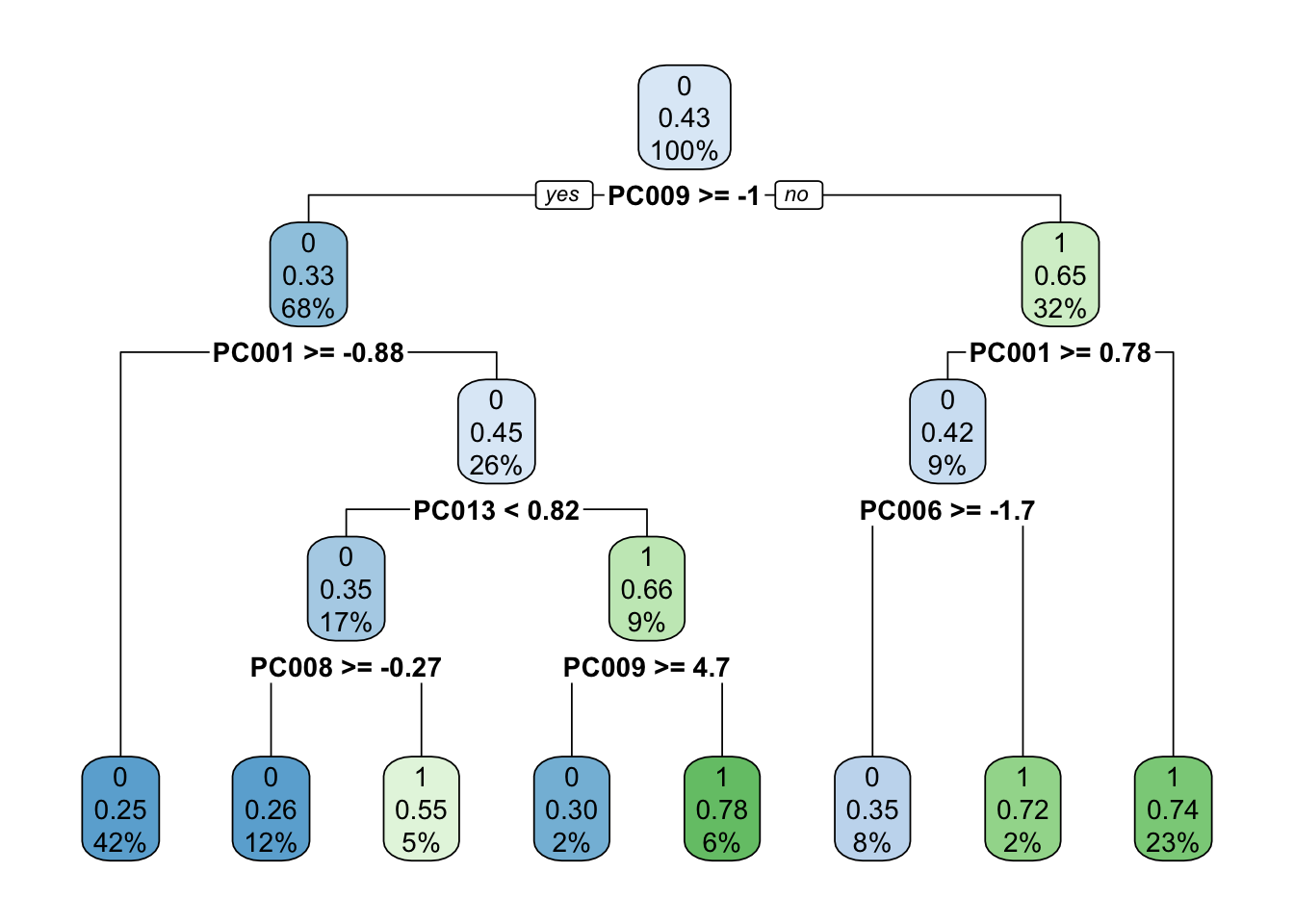Above we see the familiar plot of rpart, showing PC009 to be the most important variable.

### Gradient Boosted Trees using xgboost

Let’s build a basic boosted tree model with default values.

#### Basic

``````gbtree_spec <- boost_tree() %>%
set_engine("xgboost") %>%
set_mode("classification")

wf <- workflow() %>%
``````gbtree_fit <- wf %>%
fit(data = tweets_train)

saveRDS(gbtree_fit, "../data/nlp_with_disaster_tweets/trees/gbtree_basic_fit.rds")``````
``gbtree_fit <- readRDS("../data/nlp_with_disaster_tweets/trees/gbtree_basic_fit.rds")``

#### Tuning boosted tree parameters

Using 5-fold cross validation and a few different values of various parameters.

``````set.seed(1234)
folds <- vfold_cv(tweets_train, strata = target, v = 5, repeats = 1)

tune_spec <- boost_tree(trees = 500,
tree_depth = tune(),
learn_rate = 0.01) %>%
set_mode("classification") %>%
set_engine("xgboost")

param_grid <- grid_regular(tree_depth(), levels = 50)``````
``````doParallel::registerDoParallel(cores = parallel::detectCores(logical = FALSE))

set.seed(1234)
gbtree_grid <- tune_grid(
resamples = folds,
grid = param_grid,
metrics = metric_set(accuracy, roc_auc, f_meas),
control = control_grid(save_pred = TRUE,
verbose = TRUE)
)

saveRDS(gbtree_grid, "../data/nlp_with_disaster_tweets/trees/gbtree_grid.rds")``````
``````gbtree_grid <- readRDS("../data/nlp_with_disaster_tweets/trees/gbtree_grid.rds")

gbtree_grid %>%
collect_metrics()``````
``````## # A tibble: 45 x 6
##    tree_depth .metric  .estimator  mean     n std_err
##         <int> <chr>    <chr>      <dbl> <int>   <dbl>
##  1          1 accuracy binary     0.724     5 0.00999
##  2          1 f_meas   binary     0.778     5 0.00826
##  3          1 roc_auc  binary     0.775     5 0.00754
##  4          2 accuracy binary     0.740     5 0.0106
##  5          2 f_meas   binary     0.788     5 0.00859
##  6          2 roc_auc  binary     0.801     5 0.00668
##  7          3 accuracy binary     0.756     5 0.00862
##  8          3 f_meas   binary     0.800     5 0.00719
##  9          3 roc_auc  binary     0.813     5 0.00587
## 10          4 accuracy binary     0.761     5 0.00675
## # … with 35 more rows``````
``````gbtree_grid %>%
collect_metrics() %>%
mutate(flexibility = tree_depth,
.metric = str_to_title(str_replace_all(.metric, "_", " "))) %>%
ggplot(aes(flexibility, mean, color = .metric)) +
geom_errorbar(aes(ymin = mean - std_err,
ymax = mean + std_err), alpha = 0.5) +
geom_line(size = 1.5) +
facet_wrap(~.metric, scales = "free", nrow = 3) +
theme(legend.position = "none") +
labs(title = "Model performance against model flexibility",
subtitle = "F1-score peaks around lower flexibility values",
x = "Model flexibility i.e. tree_depth",
y = "Mean metric value")``````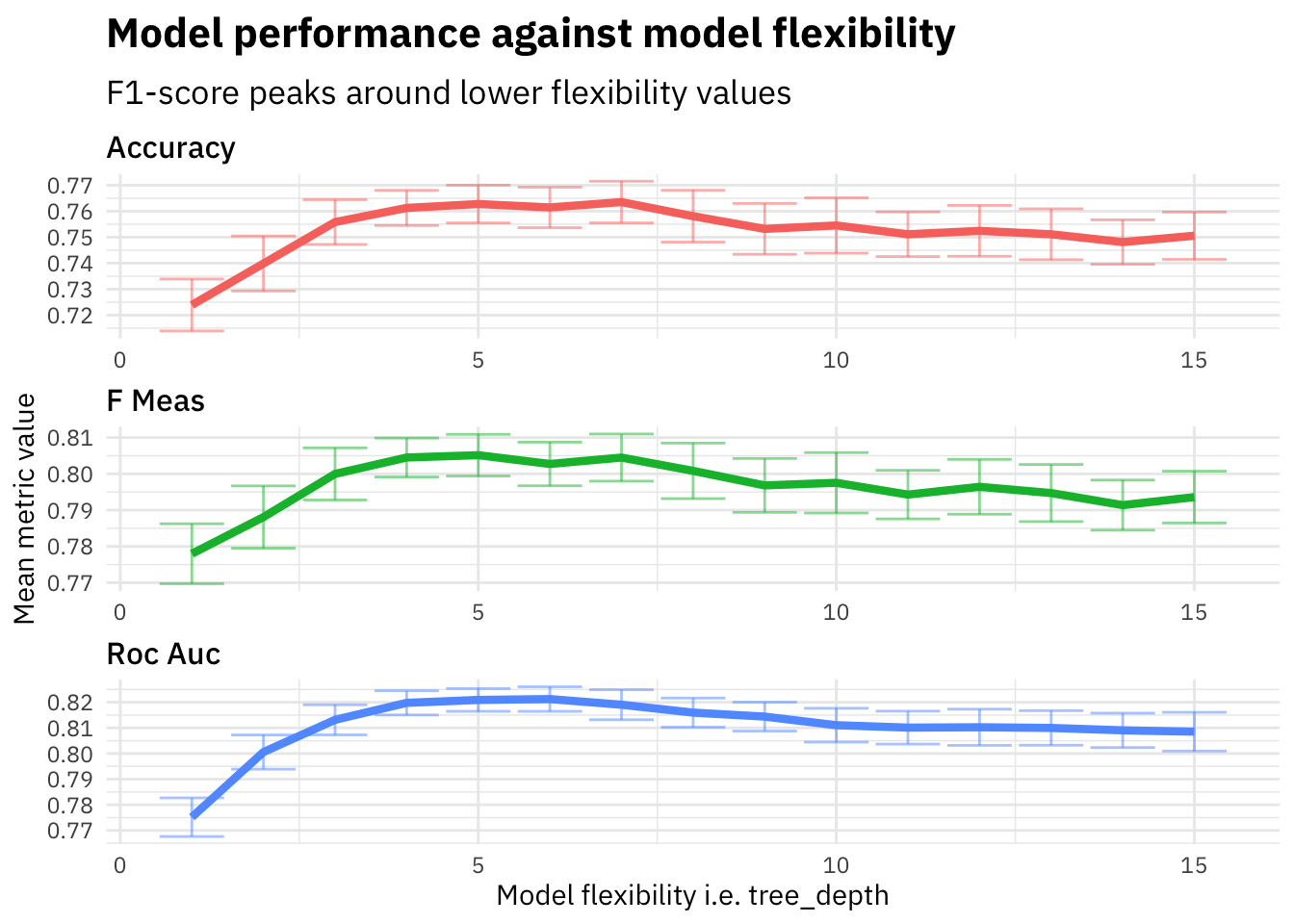As we see in the plot above, the f1-score increases on the evaluation set until around tree_depth=5 and then starts falling down. We plot the flexibility (i.e. tree_depth) to visualize how the model performance varies as the model flexibility increases. The tree model with higher tree_depth will be more flexible as it will be able to train deeper trees that try and capture more patterns in the data and might suffer from higher variance. Correspondingly, lower tree_depth will lead to a much leaner model that might suffer from bias.

Let’s pickout the best parameter tree_depth based on the highest f1-score and train our final model on the full training dataset and evaluate against cross validation dataset.

``````gbtree_grid %>%
select_best("f_meas") -> highest_f_meas

final_gbtree <- finalize_workflow(
highest_f_meas
)``````
``````last_fit(final_gbtree,
tweets_split,
metrics = metric_set(accuracy, roc_auc, f_meas)) -> gbtree_last_fit

saveRDS(gbtree_last_fit, "../data/nlp_with_disaster_tweets/trees/gbtree_last_fit.rds")``````
``````gbtree_last_fit <- readRDS("../data/nlp_with_disaster_tweets/trees/gbtree_last_fit.rds")
gbtree_last_fit %>%
collect_metrics()``````
``````## # A tibble: 3 x 3
##   .metric  .estimator .estimate
##   <chr>    <chr>          <dbl>
## 1 accuracy binary         0.764
## 2 f_meas   binary         0.811
## 3 roc_auc  binary         0.820``````

The f1-score metric on the validation set comes out to be 0.8107538 which is higher compared to all the previous models we have built in this series NLP with disaster tweets: Summary. Lets try and visualize the variable importance for different features that we used to train our model.

``````gbtree_last_fit\$.workflow[] %>%
pull_workflow_fit() %>%
vi() %>%
mutate(Importance = abs(Importance),
Variable = fct_reorder(Variable, Importance)) %>%
top_n(10, Importance) %>%
ggplot(aes(x = Importance, y = Variable)) +
geom_col() +
scale_x_continuous(expand = c(0,0)) +
labs(y = NULL,
x = "Importance",
title = "Variable Importance plot",
subtitle = "PC009 remains to be the most important feature")``````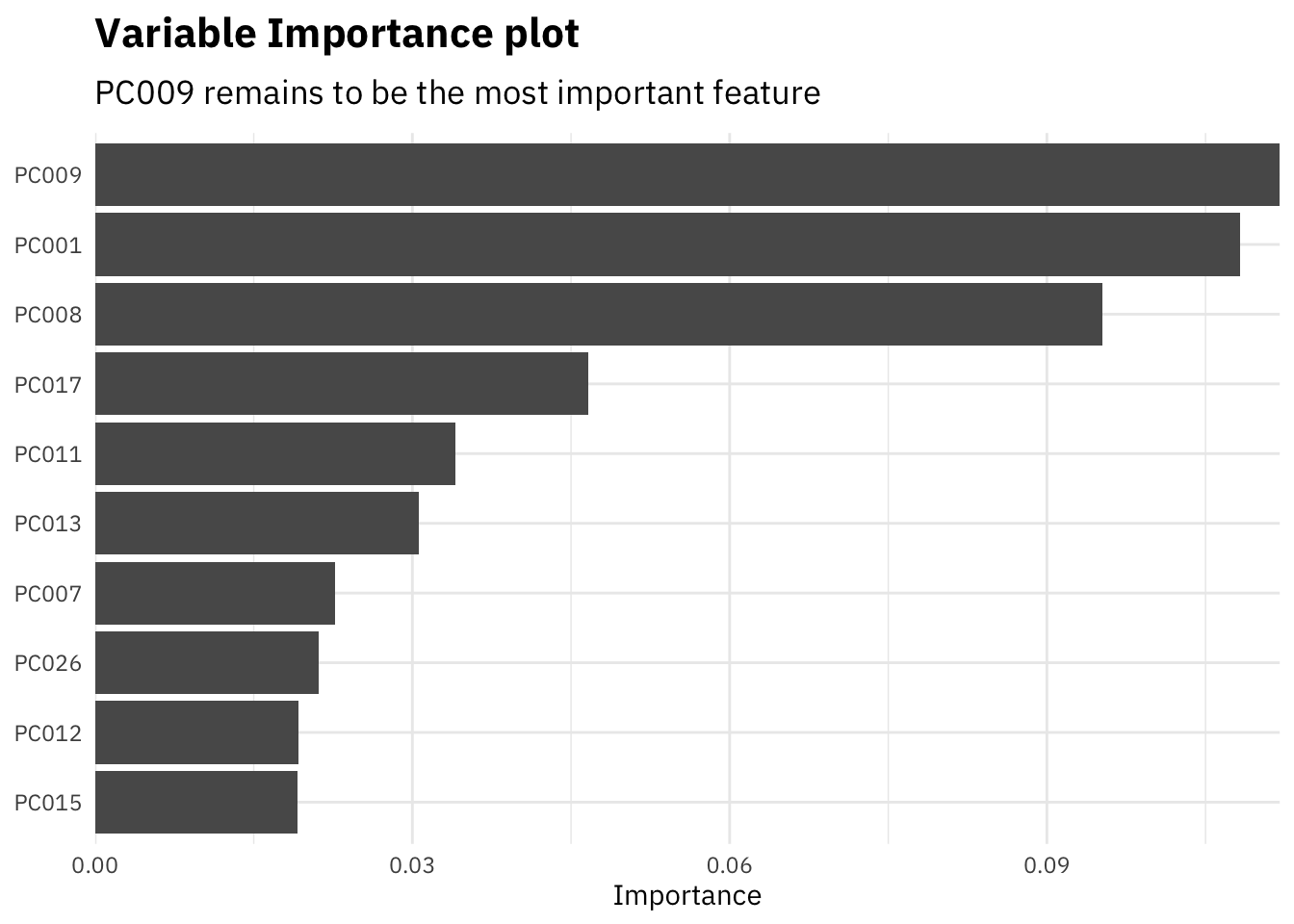Since most of our features are the dimensionally reduced different dimensions coming out of the glove embeddings, we can not interpret them. However, one thing to note here, for gradient boosted trees, none of our custom features turn out to be of any significant importance.

## Model Calibration

Another method to measure model performance is to see how well calibrated a model is on the validation dataset. Let’s compare our 3 models, gradient boosted trees here, lasso regression in Part 3 and the best k-nearest neighbor model from Part 2, by plotting calibration plots for each of them.

``````readRDS("../data/nlp_with_disaster_tweets/knn/knn_last_fit.rds") %>%
collect_predictions() %>%
mutate(pred_bucket = as.integer(`.pred_1`/(1/11))) %>%
group_by(pred_bucket) %>%
summarise(mean_truth = mean(as.numeric(as.character(target)))*100,
mean_pred = mean(`.pred_1`)*100) %>%
ungroup() %>%
ggplot(aes(x = mean_pred, y = mean_truth)) +
geom_point(color = "#F8766D", size = 3) +
geom_line(color = "#F8766D") +
scale_x_continuous(limits = c(0, 100)) +
scale_y_continuous(limits = c(0, 100)) +
geom_abline(slope = 1, intercept = 0, linetype = 2,
color = 'grey50') +
labs(x = "Mean True Prediction",
y = "Mean Truth",
title = "Calibration Plot for K-nearest neighbors model",
subtitle = "Above the diagonal signifies under-forecast")``````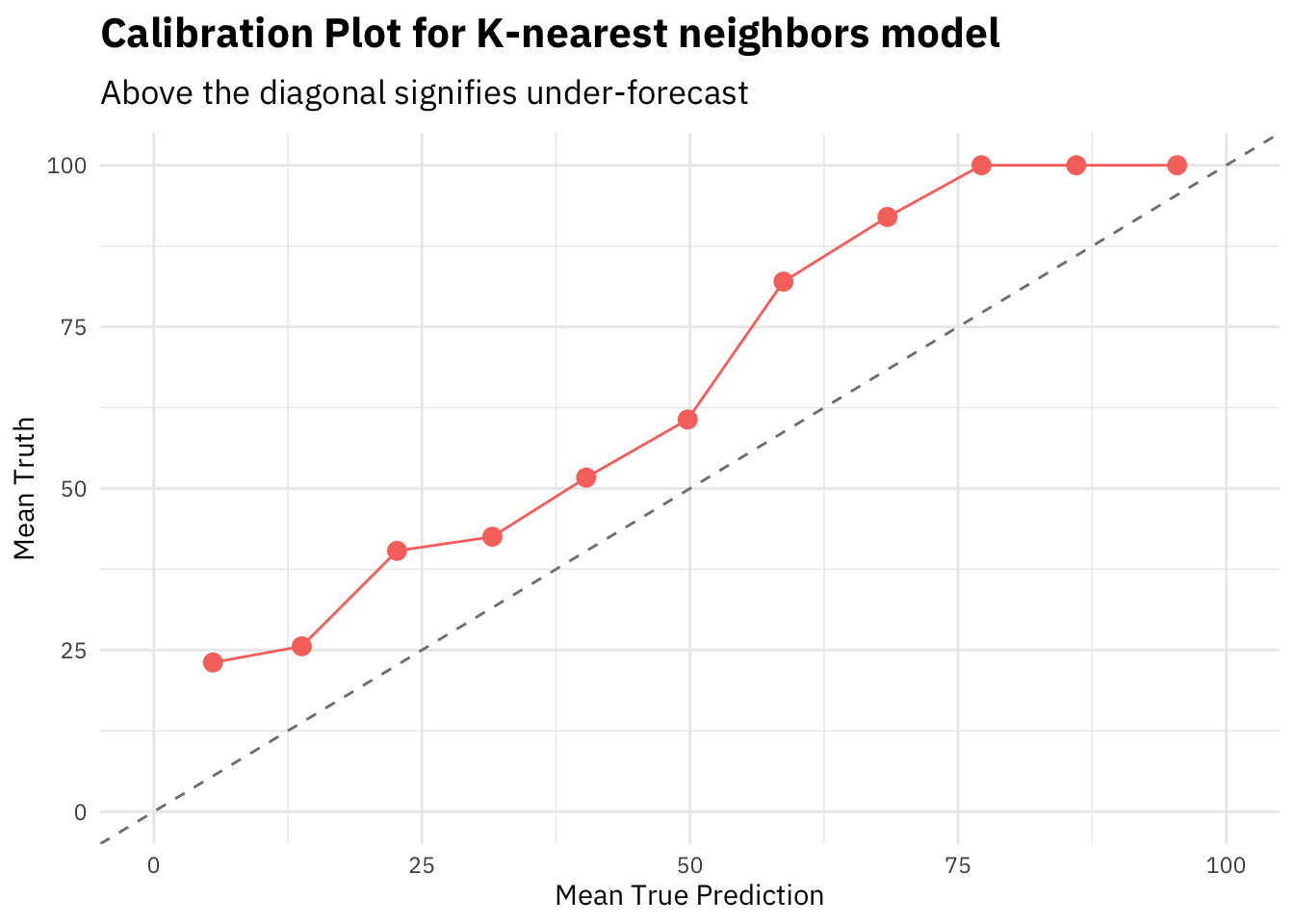``````readRDS("../data/nlp_with_disaster_tweets/glmnet/lasso_last_fit.rds") %>%
collect_predictions() %>%
mutate(pred_bucket = as.integer(`.pred_1`/(1/11))) %>%
group_by(pred_bucket) %>%
summarise(mean_truth = mean(as.numeric(as.character(target)))*100,
mean_pred = mean(`.pred_1`)*100) %>%
ungroup() %>%
ggplot(aes(x = mean_pred, y = mean_truth)) +
geom_point(color = "#F8766D", size = 3) +
geom_line(color = "#F8766D") +
scale_x_continuous(limits = c(0, 100)) +
scale_y_continuous(limits = c(0, 100)) +
geom_abline(slope = 1, intercept = 0, linetype = 2,
color = 'grey50') +
labs(x = "Mean True Prediction",
y = "Mean Truth",
title = "Calibration Plot for Lasso Regression model",
subtitle = "Close to the diagonal signifies well calibrated model")``````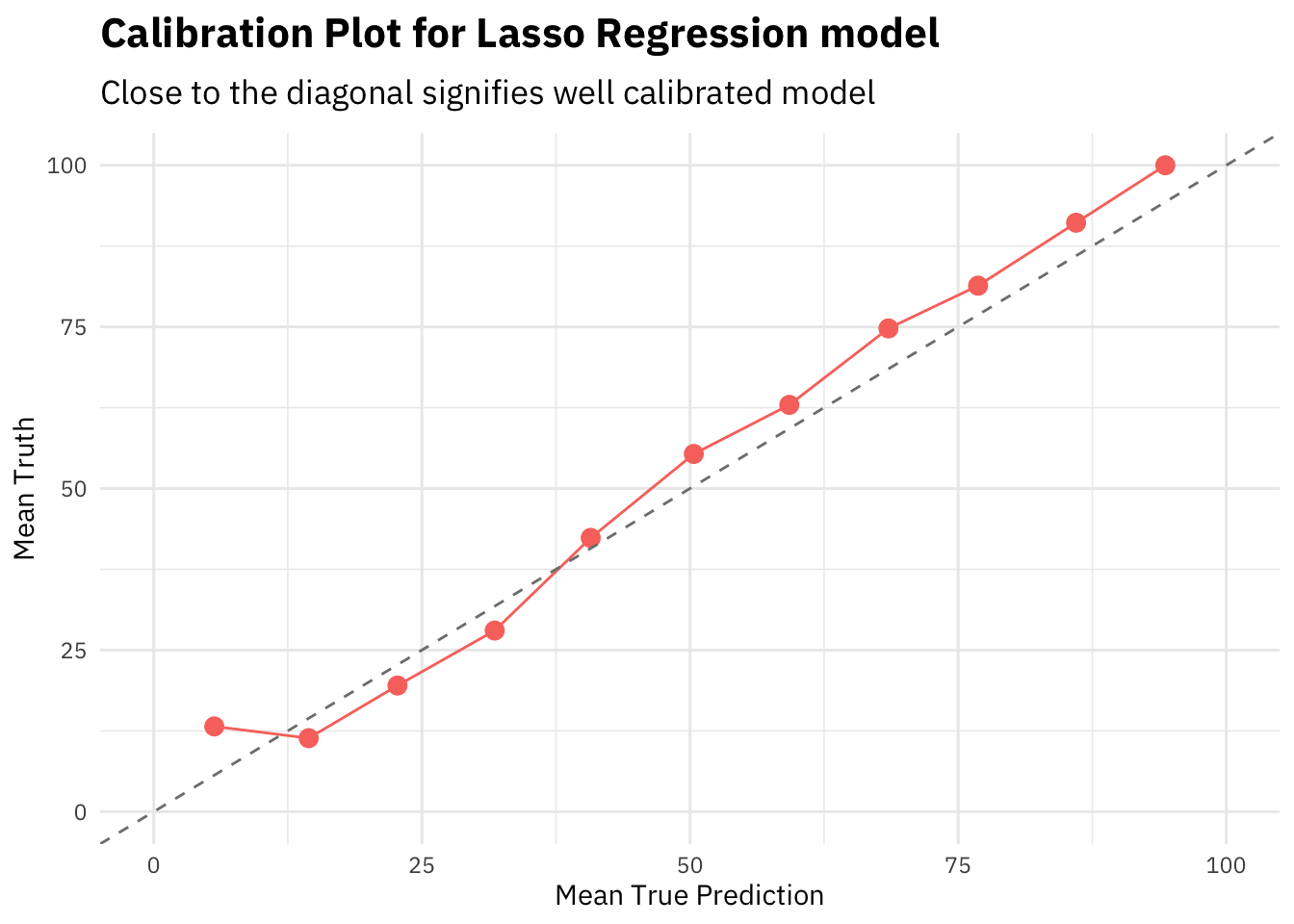``````gbtree_last_fit %>%
collect_predictions() %>%
mutate(pred_bucket = as.integer(`.pred_1`/(1/11))) %>%
group_by(pred_bucket) %>%
summarise(mean_truth = mean(as.numeric(as.character(target)))*100,
mean_pred = mean(`.pred_1`)*100) %>%
ungroup() %>%
ggplot(aes(x = mean_pred, y = mean_truth)) +
geom_point(color = "#F8766D", size = 3) +
geom_line(color = "#F8766D") +
scale_x_continuous(limits = c(0, 100)) +
scale_y_continuous(limits = c(0, 100)) +
geom_abline(slope = 1, intercept = 0, linetype = 2,
color = 'grey50') +
labs(x = "Mean True Prediction",
y = "Mean Truth",
title = "Calibration Plot for Gradient Boosted Tree model",
subtitle = "Close to the diagonal signifies well calibrated model")``````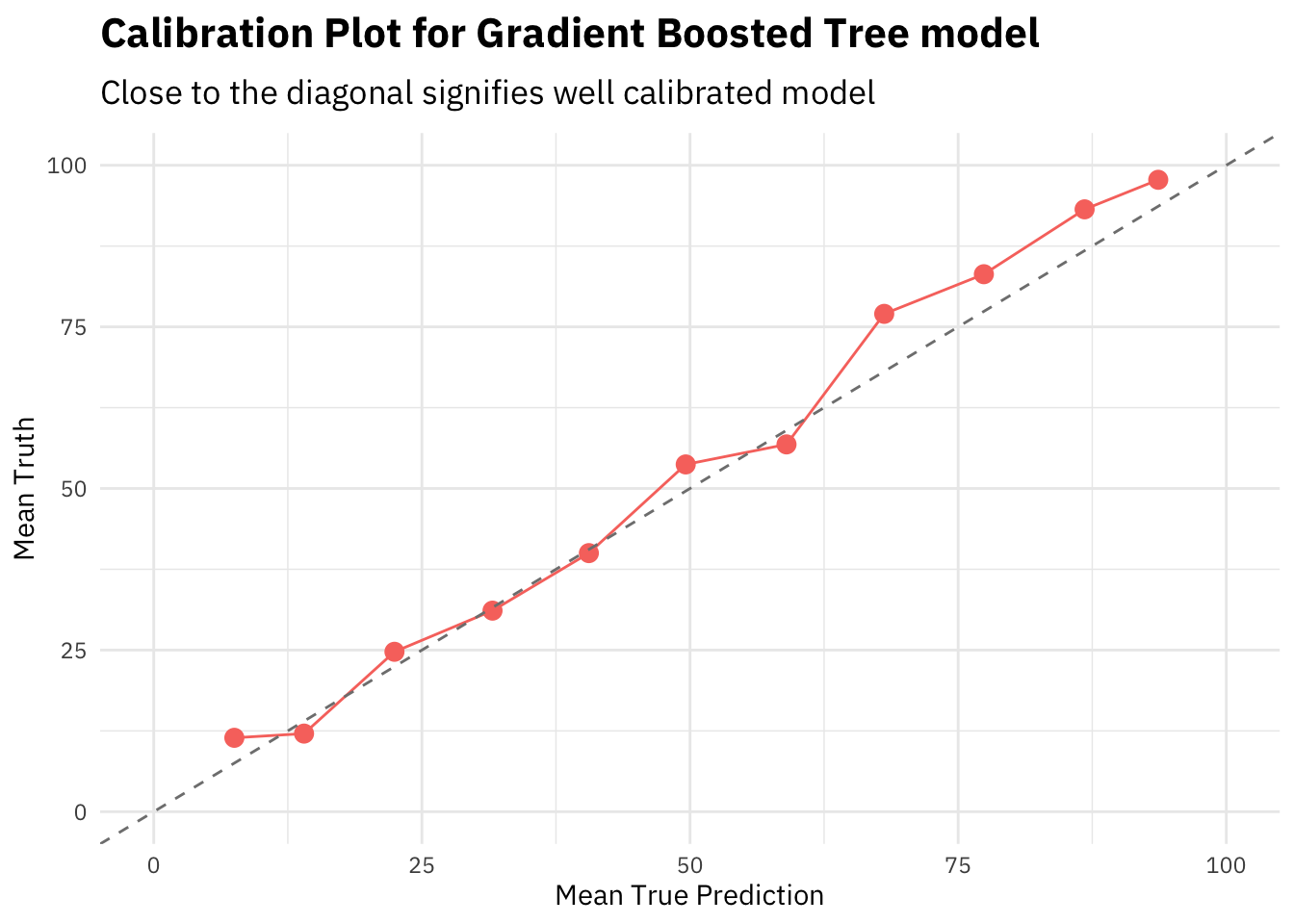In general, closer the calibration curve to the diagonal, the better. When a model is well calibrated, the mean prediction probability will be equal to mean truth. Meaning the model is predicting the target in the same ratios as the actual truth values.
The KNN model calibration plot shows that the curve is above the diagonal, i.e. the model is frequently under estimating the predicted probabilities.
However, both lasso regression and gradient boosted tree models seem to be well calibrated out of the box. That’s a sign of good stable algorithm and training procedure.
Note that all the predicted probabilities here are calculated on the cross validation set.

# Summary

In this part I built tree based models in order to understand how the problem space can be modelled with these approaches. We do see a small improvement in f1-score as compared to all our previous approaches. Note that, due to limited compute resources on my mac, I have kept the size of tuning grid to be small. In the next part of this series, I will move focus towards neural networks based models and see how “deep” we can go. Other NLP strategies like Named-entity recognition and others are also on my bucket list for this series.